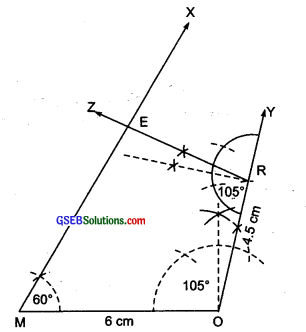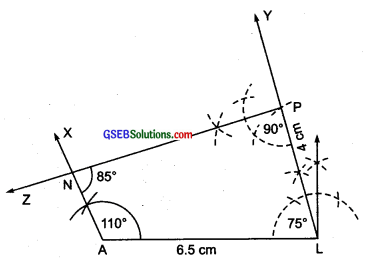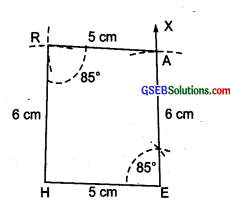# GSEB Solutions Class 8 Maths Chapter 4 Practical Geometry Ex 4.3

Gujarat Board GSEB Textbook Solutions Class 8 Maths Chapter 4 Practical Geometry Ex 4.3 Textbook Questions and Answers.

## Gujarat Board Textbook Solutions Class 8 Maths Chapter 4 Practical Geometry Ex 4.3

Question 1.
MORE
MO = 6 cm
OR = 4.5 cm
∠M = 60°
∠O = 105°
∠R = 105°PLAN
PL = 4 cm
LA = 6.5 cm
∠P = 90°
∠A = 110°
∠N = 85°

(iii) Parallelogram
OKAY
HE = 5 cm
EA = 6 cm
∠R = 85°(iv) Rectangle
HEAR
OK = 7 cm
KA = 5 cm
Solution:
(i) Steps of constructions:
I. Draw a Line segment MO = 6 cm
II. At M, draw $$\overrightarrow { MX }$$ , such that ∠OMX = 60°
III. At O, draw $$\overrightarrow { OY }$$ , such that ∠MOY = 105°
IV. From $$\overrightarrow { OY }$$ , cut off OR = 4.5 cm
V. At R, draw $$\overrightarrow { RZ }$$ , such that ∠ORZ = 105°
Let $$\overrightarrow { RZ }$$ intersects $$\overrightarrow { MX }$$ at E.Thus, MORE is the required quadrilateral.

(ii) Steps of construction:
I. Draw a line segment AL = 6.5 cm.
II. At A, draw $$\overrightarrow { AX }$$ such that ∠LAX = 110°
III. At L, draw $$\overrightarrow { LY }$$ such that ∠ALY = 75°.
Note: ∠L = 75° is not given, but we can determine it using angle sum property
∵ Sum of three given angles
= 110° + 90° + 85° = 285°
∴ The fourth angle ∠L = 360° – 285° = 75°IV. rom $$\overrightarrow { LY }$$ , cut-off LP = 4 cm.
V At P, draw $$\overrightarrow { PZ }$$ such that ∠LPZ = 90°
Let $$\overrightarrow { PZ }$$ and $$\overrightarrow { AX }$$ intersect at N.
Thus, PLAN is the required quadrilateral.(iii) Steps of construction:
I. Draw a line segment $$\overrightarrow { HE }$$ = 5 cm.II. At E, draw $$\overrightarrow { EX }$$ such that ∠HEA = 85°.
III. From $$\overrightarrow { EX }$$ , cut-off EA = 6 cm.
IV. With centre at A and radius = 5 cm, draw an arc towards H.
V With centre at H and radius = 6 cm, draw an arc such that it intersects the previous arc at R.
VI. Join RA and RH.
Thus, HEAR is the required parallelogram

(iv) Steps of construction:I. Draw a line segment Ok = 7 cm
II. draw $$\overrightarrow { OP }$$ such that ∠KOP = 90°.
III. From $$\overrightarrow { OP }$$ , cut-off $$\overrightarrow { OY }$$ = 5 cm.
IV. At K, draw $$\overrightarrow { KQ }$$ such that ∠OKQ = 90°.
V. From $$\overrightarrow { KQ }$$ cut-off KA = 5 cm.
VI. Join A and Y.
Thus, OKAY is the required rectangle